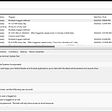# A walk-through Python Code Optimization:

Previous lectures: Episode 1 Decimal and Binary notation

Picking up where we left off last time, we are going to explain how to rewrite numbers from base 10 to base 2, and how generalize that expression in order to rewrite those decimal numbers to different bases, and of course, we’ll explain briefly how bases aside of base 2 and base 10 are made. Finally, we’ll make an algorithm which can make that for us, and in further episodes we’ll evaluate the algorithm optimization.

Before we start, I’d like to emphasise: the numbers we write in different bases are the same numbers but expressed in a different way.

Then, recalling our last equation:

From now on, we’d assume that we all have read the previously episode, therefore we’ll go straight to the point — or at least I’ll try it –.

If we would like to rewrite (15)10 into another base, the first step we shall do is to divide the number, 15, by the base number I want to convert it, in this particular case, we are going to work with base 2, so we’re going to divide it by 2. If we were trying to convert it to base, for instance, 5, we’ll divide 15 by 5, so the divisor will always be the number of the base to which we want to rewrite the decimal number, and until we finish, it won’t change. And as we all really like the famous Equation 2, we’ll express all the divisions from this point on like that, but, before we start, lets clarify a little bit of notation that I may have left out to explain in our last episode.

When we refer to a number in base 10, we’ll put that number between parentheses, and in the bottom right side of the right parentheses, we’ll indicate the base we’re expressing the number — or what’s the same, a subscript after the last parentheses with the base number–. Let’s take a look:

And the same with binary or other bases:

And that’s for simple convention, but we better not forget it, because it can be the case that two different decimal numbers have the same representation in two different bases. And at the end we’ll extend that concept, once we now more about other bases.

So, if we’d like to rewrite decimal 15 to base 2, we are going to divide it by 2, for that particular example we’ll see the “normal” division beside equation:

Now, we could express the above equation in a different way that will keep the meaning.

That’s because the number 1 it’s the neutral element from a multiplication, as 0 it’s the neutral element for the sum. Think about it, what do we get if we sum 6 plus 0? Of course, 6! And if we multiply 6 times 1? Yes, you’ve hit the target, also 6!

Now, we can also rewrite the last equation into the following, and if you can’t recognize where it comes from, take a look on the 7th (Base 2 slots notation) image form the previous episode.

Once we’ve done the first division, we’ll continue dividing our quotient, in this case 6, and we’ll as well express it in the power form.

We’ll keep repeating the process of dividing the quotient of the division until our quotient becomes 0.

To simplify a bit, we could perfectly get rid of the first term on the right hand of our last equation, because we’ve reached 0 as the quotient.

Once we have expressed all the quotients like we’d express in Equation 2, we can start building the real equation, and to do that, we have to substitute from the bottom up with the equivalent base 2 representation. In other words, get the right hand of the last equation and substitute the coloured number from the above equation with it.

We’ll do exactly the same process until we reach our first equation where our dividend was 13.

Finally:

That’s the trickiest part and we’ve explained the mathematical approach, but don’t worry too much about the maths, you can go through without fully understanding how to convert the decimal numbers to binary by substituting the equations, every person has their own way to understand the things and I’m sure you’ll understand it your way!

The important thing here is the PATTERN. We can notice that every time that the division has a reminder, it means that our binary notation would have a 1 in that specific position. Let me explain that concept further:

When we divide the dividend by the base (the divisor), we are going to keep track of the number of times we’ve made a division beginning from 0 (first division will be count = 0). In every division, we multiply the reminder times 2 to the power of the counter we’ve tracked. We’ll keep doing the divisions until we reach 0 as quotient.

Let’s look at a picture of what we mean with this:

And if we put it in the “slots” that we saw in the previous lecture, we’ll have:

Exactly the same expression that we reached above, substituting the equations.

For those who are more interested in the maths, I am going to let you in the next paragraph another example of conversion with a bigger decimal number.

We are going to rewrite the decimal number (175)10 to a binary notation. In this particular example we won’t make it only with those 4 “slots” that we had. And as we saw in the decimal example on Episode 1, we’ll add more “slots” until we can represent our number in a binary notation, and when we reach 0 as a quotient, we can give the process as finished.

If we, carefully, substitute from bottom to top the right part of the equation into the above equation, which the number is remarked, we’ll end with something like this. Keep in mind, that also in this equation, we’ve got rid of the first term of the last equation.

And if we carefully go from inside the parentheses to outside applying the distributive property, we’ll end with:

And as in the other example, if we take count of the divisions and multiply the reminder times 2 to the counter power:

Now, before we make an algorithm of conversion, we are going to see which other bases are, and we can see all types of bases. The biggest we could see is base 62, and we’ll discuss that later, but normally we use until base 16, hexadecimal. And how can we represent numbers in bases bigger than 10?

To summarize, the base number restricts us from using numbers above, and including, the number base. If we want to write numbers, for example, in base 5, the bigger number we can use in the “slots” is 4, remember that we still have the 0. So, for base 8 we can only use until the number 7. And for those bases bigger than base 10? Umm… have you ever seen a deck of poker cards?

It’s nearly the same system! In a poker deck we have card numbers up to 10, being 1 the first card (in reality for those who aren’t familiar with the deck, it’s called As Card, and instead of 1, the card has the letters “A” and “s” and can represent either the smaller value or the biggest). Above the tenth card, we find the cards “J”, “Q” and “K”, which each one is greater than the previous. And would represent the eleventh, twelfth and thirteenth in a numerical value.

With the bases, we deal with the problem of number representation in the same way, we assign some symbol to the numbers above 9. For instance, the undecimal system (base 11) uses the numbers from 0 to 9 and, to represent the number 10, it uses an “A”. The ASCII letters are normally used, in an alphabetical order, to represent the numbers above 9. If we only use the abecedary with capital letters, we’ll have up to base 36, and if we also use the abecedary letters in lower case, will have until base 62.

Therefore, we’ll have the following encoding for bases.

“0123456789ABCDEFGHIJKLMNOPQRSTUVWXYZabcdefghijklmnopqrstuvwxyz”

Where the process of encoding is; once we have the remainder number, count in the above encode string and get the symbol (number or letter) that it’s in the number position of that reminder.

Of course, we can use more symbols to represent above base 62, like: !, ¡, ¿, ?, |, /… And we could use any order in our encoding system to represent the numbers above 9, but we shall always have a table or a list showing which number corresponds to each symbol.

Now that we know how the bases are made, we’re going to see an example to show why the parentheses nomenclature is so important.

As we said at the beginning, we can find different decimal numbers with the same number in different bases.

You can see below, the rebase from base 10 algorithm. There are many different approaches to reach our goal, that’s one of the cool things from programming, but in this particular example, I have extended the code as much as I could. There are already functions that make some of the process faster, but as the goal of all these articles is Python code optimization, in further episodes I’ll explain different approaches to that algorithm, and we’ll make some velocity test.

That’s all for today, in the next episode, Episode 3 — I still have to think ofa title — we’ll see, this time for real, what are actually those slots, and what everything we’ve explained has to do with computers memory. I hope you’ve understood everything we’ve explained and feel free to ask if you have some doubts.

--

--

--

## More from Alex Abades Grimes

“If you can’t explain it simply, you don’t understand it well enough” -Albert Einstein.

Love podcasts or audiobooks? Learn on the go with our new app.

## Explore the Activity Life Cycle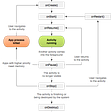## *Open Source at AWS: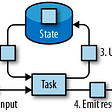## The Interview Process: What to Expect When You Apply to YLD## Ethereum 2.0 Casper+Sharding Development Update #14 — Prysmatic Labs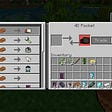## What is Google Cloud Function and How to write a program for it.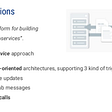“If you can’t explain it simply, you don’t understand it well enough” -Albert Einstein.

## A practical approach to Software Development Lifecycle… Build your first Python package!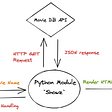## Scheduling Python Scripts using Azure Function App and Task Scheduler — Part 1